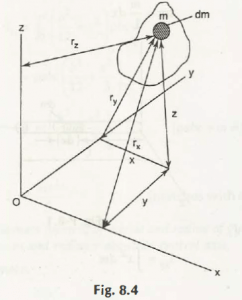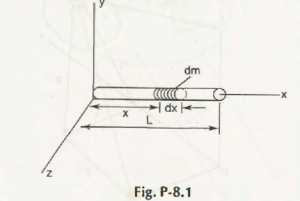Consider the Fig. 8.4,Let the mass moment of inertia about x- axis be Ixx

Mass moment of inertia about y- axis be Iyy

Mass moment of inertia about z- axis be Izz

So, it can be written as-

Ixx = x2 dm = 2 + z2) dm

Iyy = y2 dm = 2 + z2) dm

Izz = z2 dm = 2 + y2) dm

Suppose z is negligible as compare to x and y, then z = 0

Hence,

Ixx2 dm

= 2 x pt dA

= pt 2 dA

1. Ix

Similarly,

Iyy = pt. Iy

Now,

Ixx + Iyy = pt (Ix + Iy)

= pt. Iz

Hence,

Ixx + Iyy = Izz

Considering the above Equations, 8.8 is for thin flat plate and whenever we calculate differential mass dm for element of thickness dz

Thus, it can be modified as-

dIxx + dIyy = dIzz

Example 8.1:

Suppose a slender rod of length L, mass m which is perpendicular to the axis where x-axis is passes through it as shown in Fig. P-8.1.Find its mass moment of inertia

Solution:

Take, dm = m/ L dx

Thus,

The mass moment of inertia about y-axis can be calculated as,

Iyy2 dm

= m/ L x 1/ 3 x L3

= mL2/ 3

Links of Next Mechanical Engineering Topics:-### Customer Reviews

My Homework Help
Rated 5.0 out of 5 based on 510 customer reviews at